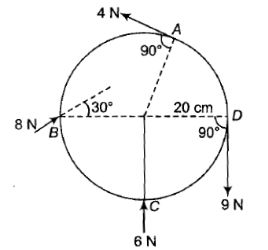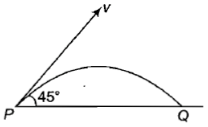A solid sphere rolls without slipping down a $30°$ inclined plane. If g = 10 $\mathrm{m}/{\mathrm{s}}^{2}$, the acceleration of the rolling sphere is

1.  5 ${\mathrm{ms}}^{-2}$

2.  $\frac{7}{25}{\mathrm{ms}}^{-2}$

3.  $\frac{25}{7}{\mathrm{ms}}^{-2}$

4.  $\frac{15}{7}{\mathrm{ms}}^{-2}$

Concept Questions :-

Rolling motion
High Yielding Test Series + Question Bank - NEET 2020

Difficulty Level:

If the equation for the displacement of a particle moving on a circular path is given by $\theta =2{t}^{3}+0.5$, where $\theta$ is in radian and t is in second, then the angular velocity of the particle after 2s is

Concept Questions :-

Rotation motion : Introduction
High Yielding Test Series + Question Bank - NEET 2020

Difficulty Level:

A pan containing a layer of uniform thickness of ice is placed on a circular turntable with its centre coinciding with the centre of the turn table. The turntable is now rotated at a constant angular velocity about a vertical axis passing through its centre and then driving is withdrawn. There is no friction between the table. As the ice melts

1.  the angular velocity of the system decreases

2.  the angular velocity of the system increases

3.  the angular velocity of the system remains unchanged

4.  the moment of inertia of the system decreases

Concept Questions :-

Angular momentum
High Yielding Test Series + Question Bank - NEET 2020

Difficulty Level:

The motion of planets in the solar system is an example of the conservation of

1.  mass

2.  Linear momentum

3.  Angular momentum

4.  Energy

Concept Questions :-

Angular momentum
High Yielding Test Series + Question Bank - NEET 2020

Difficulty Level:

A circular disc is to be made by using iron and aluminium, so that it acquires maximum moment of inertia about its geometrical axis.  It is possible with

(1) iron and aluminium layers in alternate order

(2) aluminium at interior and iron surrounding it

(3) iron at interior and aluminium surrounding it

(4) either '1' or '3'

High Yielding Test Series + Question Bank - NEET 2020

Difficulty Level:

A particle of mass 15 kg has an initial velocity m/s. It collides with another body and the impact time is 0.1 s, resulting in a velocity m/s after impact. The average force of impact on the particle is

1.

2.

3.

4.

Concept Questions :-

Linear momentum
High Yielding Test Series + Question Bank - NEET 2020

Difficulty Level:

Two Circular discs A and B are of equal masses and thicknesses but made of metal with densities (). If their moments of inertia about an axis passing through their centers and perpendicular to circular faces be , then

1.

2.

3.  ${\mathrm{I}}_{\mathrm{A}} < {\mathrm{I}}_{\mathrm{B}}$

4.

Concept Questions :-

Moment of inertia
High Yielding Test Series + Question Bank - NEET 2020

Difficulty Level:

A body is rolling without slipping on a horizontal surface and its rotational kinetic energy is equal to the translational kinetic energy. The body is

1.  Disc

2.  Sphere

3.  Cylinder

4.  Ring

Concept Questions :-

Moment of inertia
High Yielding Test Series + Question Bank - NEET 2020

Difficulty Level:

A wheel of radius 20 cm forces applied to it as shown in the figure. The torque produced by the forces 4 N at A, 8 N at B, 6 N at C at D at angles indicated is1.  5.4 N-m anticlockwise

2.  1.80 N-m clockwise

3.  2.0 M-m clockwise

4.  3.6 n-m clockwise

Concept Questions :-

Torque
High Yielding Test Series + Question Bank - NEET 2020

Difficulty Level:

A projectile of mass m is fired with velocity v from a point P, as shown. Neglecting air resistance, the magnitude of the change in momentum between the points P and arriving at Q is1.  Zero

2.  $\frac{\mathrm{mv}}{\sqrt{2}}$

3.

4.  2 mv

Concept Questions :-

Linear momentum